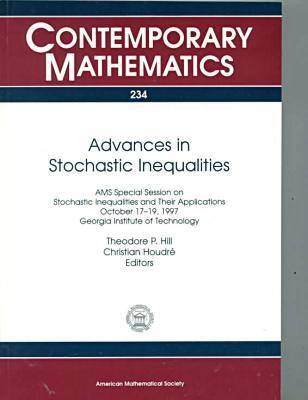•# Advances in Stochastic Inequalities: AMS Special Session on Stochastic Inequalities and Their Applications, October 17-19, 1997, Georgia Institute of Technology - Contemporary Mathematics (Paperback)

£55.50
Paperback 212 Pages / Published: 30/05/1999
• We can order this

Usually dispatched within 3 weeks

This volume contains 15 articles based on invited talks given at an AMS Special Session on 'Stochastic Inequalities and Their Applications' held at Georgia Institute of Technology (Atlanta). The session drew international experts who exchanged ideas and presented state-of-the-art results and techniques in the field. Together, the articles in the book give a comprehensive picture of this area of mathematical probability and statistics.The book includes new results on the following: convexity inequalities for ranges of vector measures; inequalities for tails of Gaussian chaos and for independent symmetric random variables; Bonferroni-type inequalities for sums of stationary sequences; Rosenthal-type second moment inequalities; variance inequalities for functions of multivariate random variables; correlation inequalities for stable random vectors; maximal inequalities for VC classes; deviation inequalities for martingale polynomials; and, expectation equalities for bounded mean-zero Gaussian processes. Various articles in the book emphasize applications of stochastic inequalities to hypothesis testing, mathematical finance, statistics, and mathematical physics.

Publisher: American Mathematical Society
ISBN: 9780821810866
Number of pages: 212
Weight: 397 g
Dimensions: 337 x 178 mm